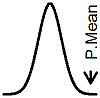P.Mean: BUGS model for the simple Poisson accrual model (created 2012-04-18). News: Sign up for "The Monthly Mean," the newsletter that dares to call itself average, www.pmean.com/news.I have been working on various extensions to the Bayesian model for patient accrual. Most of these extensions would require the use of the program BUGS. The first step to developing these extensions is to program simple models in BUGS, models where there is a closed form analytical solution. Here is an example of using BUGS to model the simple Poisson accrual model.

```ac.model <- function() {  k <- n*P  V <- T*P  lambda ~ dgamma(k,V)  theta <- 1 / lambda  for (i in 1:tm) {   count[i] ~ dpois(lambda)  }  t.rem <- T-tm  lambda.t <- lambda*t.rem  n.rem ~ dpois(lambda.t)  n.tot <- sum(count[])+n.rem `````` } writeModel(ac.model,"PoissonAccrualModel.txt")  ac.data <- list(n=350,T=1095,P=0.5,tm=240, count=c(1,0,1,0,0,0,0,0,0,0,0,0,0,0,0,0,0,  0,0,0,0,0,0,0,0,0,0,0,1,0,0,0,0,0,0,0,0,0,  0,0,0,0,0,0,0,0,0,0,0,0,0,0,0,0,0,0,0,1,1,  0,1,0,0,0,0,0,0,0,0,0,0,1,0,0,0,0,0,0,0,2,  0,0,0,0,1,0,0,0,0,0,0,0,0,0,1,1,0,0,0,1,0,  0,0,0,0,0,0,0,1,0,0,0,0,0,0,0,0,0,0,0,1,0,  0,1,0,0,0,0,0,1,0,0,0,0,0,0,1,0,0,0,0,0,0,  0,1,0,0,0,0,0,0,0,0,0,0,0,1,1,0,0,0,0,0,0,  0,1,0,0,0,0,1,0,0,0,0,0,0,2,0,1,0,0,0,0,0,  0,0,1,0,0,1,0,1,1,2,0,0,0,1,0,0,0,0,0,0,0,  1,1,0,0,0,0,0,0,0,1,0,0,0,0,0,0,0,0,0,0,0,  2,0,0,0,0,0,1,1,0,0,0,0,1)) bugsData(ac.data,"PoissonAccrualData.txt") ac.init <- list(list(lambda=1/3,n.rem=300)) bugsInits(ac.init,numChains=1,"PoissonAccrualInits.txt") modelCheck("PoissonAccrualModel.txt") modelData("PoissonAccrualData.txt") modelCompile() modelInits("PoissonAccrualInits.txt") modelUpdate(1000) samplesSet(c("theta","n.tot")) modelUpdate(1000) samplesStats("*") m <- sum(count) k <- m+n*P V <- tm+T*P p <- c(0.025,0.5,0.975) `````` c1 <- 350-41 c2 <- 350*0.5+41 c3 <- 1095*0.5+239 qgamma(p,c2,c3) bpct <- qbeta(p,c1,c2) c3*bpct/(1-bpct) c3*c1/c2*qf(p,2*c1,2*c2)```

Here are some comments on the program.

It's easy to derive the closed form solutions for the Poisson accrual case. Let n represent the number of patients that you hope to recruit and T represent the time in days that you hope the recruitment will take.Assume that the number of patients recruited on day i (i=1,...,m) follows a Poisson distribution with rate parameter lambda.Place a gamma prior on lambda.The strength of the prior belief is controlled by P, a number between zero and one. Let k=nP and V=TP. The posterior distribution for lambda is proportional to the product of the prior distribution and the likelihood.which simplifies toOnce you figure out the right normalizing constant, you will establish that the posterior distribution is gamma with k=nP+m and V=TP+tm. Now you want the posterior predictive distribution for the number of patients recruited during the remaining T-tm days in the trial. If lambda were known, then the number of patients recruited for the remaining T=tm would be Poisson, but you have to account for the uncertainty in the rate parameter. You do this by integrating the product of the Poisson probability and the posterior.Note the extra multiplier of (T-tm) in the Poisson distribution. You need to predict not a the Poisson count for a single day but the sum of Poisson counts across the remaining T-tm days in the study. Pull everything out of the integral that does not depend on lambda.When you find the right normalizing constants, this simplifies towhich is a negative binomial distribution. To see this, setandThen you getThis page was written by Steve Simon and is licensed under the Creative Commons Attribution 3.0 United States License. Need more information? I have a page with general help resources. You can also browse for pages similar to this one at Accrual Problems.